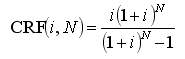# HOMER Pro 3.10The capital recovery factor is a ratio used to calculate the present value of an annuity (a series of equal annual cash flows). The equation for the capital recovery factor is:where: i N = number of years

Example: For i = 7% and N = 5 years, the capital recovery factor is equal to 0.2439. A \$1000 loan at 7% interest could therefore be paid back with five annual payments of \$243.90. The present value of the five annual payments of \$243.90 is \$1000.